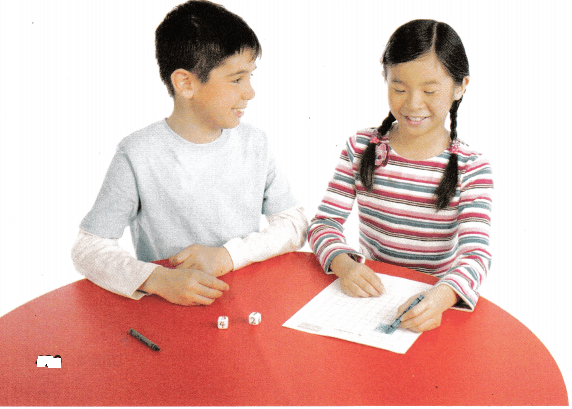# Texas Go Math Grade 3 Unit 4 Answer Key Geometry and Measurement

Refer to our Texas Go Math Grade 3 Answer Key Pdf to score good marks in the exams. Test yourself by practicing the problems from Texas Go Math Grade 3 Unit 4 Answer Key Geometry and Measurement.

## Texas Go Math Grade 3 Unit 4 Answer Key Geometry and Measurement

Show What You Know

Check your understanding of important skills.

Sort Two-Dimensional Figures by Attributes

Question 1.
Circle the figures with 4 sides and 4 vertices.Explanation:
A rectangle, square, trapezoid, and a rhombus have 4 sides and 4 vertices

Model Multiplication with Arrays

Use the array. Complete the multiplication sentence.

Question 2.__________ × ___________ = __________
3 x 4 = 12
Explanation:
Number of rows x number of columns

Question 3.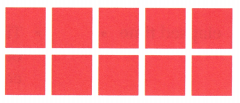_________ × ___________ = ___________
Answer: 2 x 5 = 10
Explanation:
Number of rows x number of columns

Tell Time to the Minute: Write the time.

Question 4.Explanation:
The hours hand points in between 8 and 9
the minute hand points between 4 and 5

Question 5.Explanation:
The hours hand points in between 7 and 8
the minute hand points between 1 and 2

Question 6.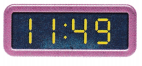Explanation:
The hours hand points in between11 and 12
the minute hand points between 9 and 10

Vocabulary Builder

Visualize It

Complete the tree map by using the words with a ✓.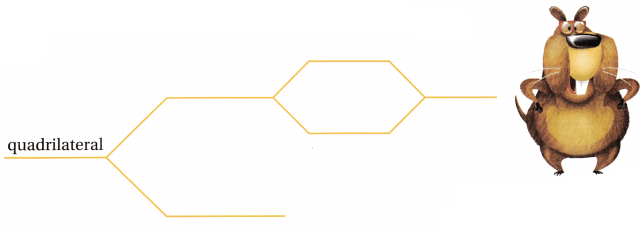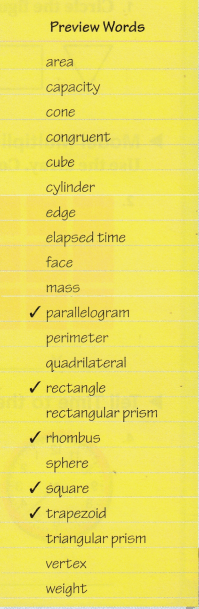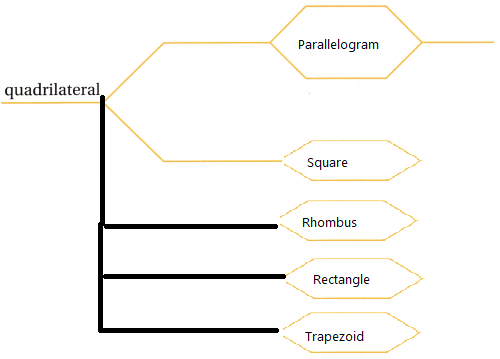Explanation:
The tree is completed with preview words.

Understand Vocabulary

Draw a line to match the word with its definition.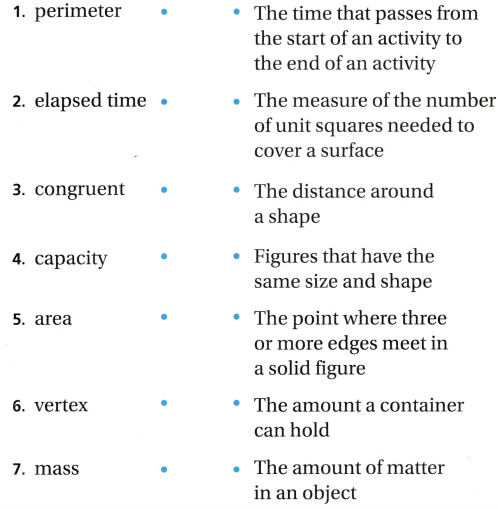Explanation:
Written the meaning for the words

Vocabulary

When you find how long or tall something is, you find its length. When you find how much matter something has, you find its mass.

Use the words in the Word Bank to complete the table below.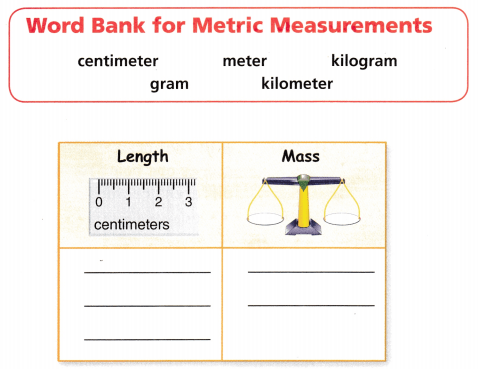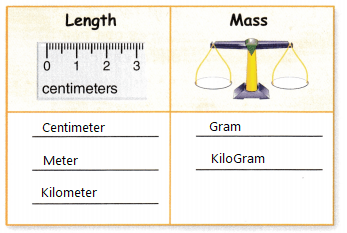Writing Look at the units of mass you listed. Write them in order by size from smallest to largest.Explanation:Written them in order by size from smallest to largest.

Reading Look for this book in your library. Counting On Frank, by Rod Clement.

Area in Action

Object of the Game To make as many rectangles with a given area as possible.

Materials

• 2 number cubes labeled 1-6
• 1-centimeter grid paper
• crayons

Number of Players 2

How to Play

(1) Player 1 tosses the number cubes. The sum of the numbers tells the area, in square units, that Player 1 should color.

(2) Player 1 uses crayons to show the different rectangles that can be made with that area. Player 1 scores one point for every different rectangle made.

(3) Player 2 repeats Steps 1-2.

(4) The player with the most points after 3 rounds wins.